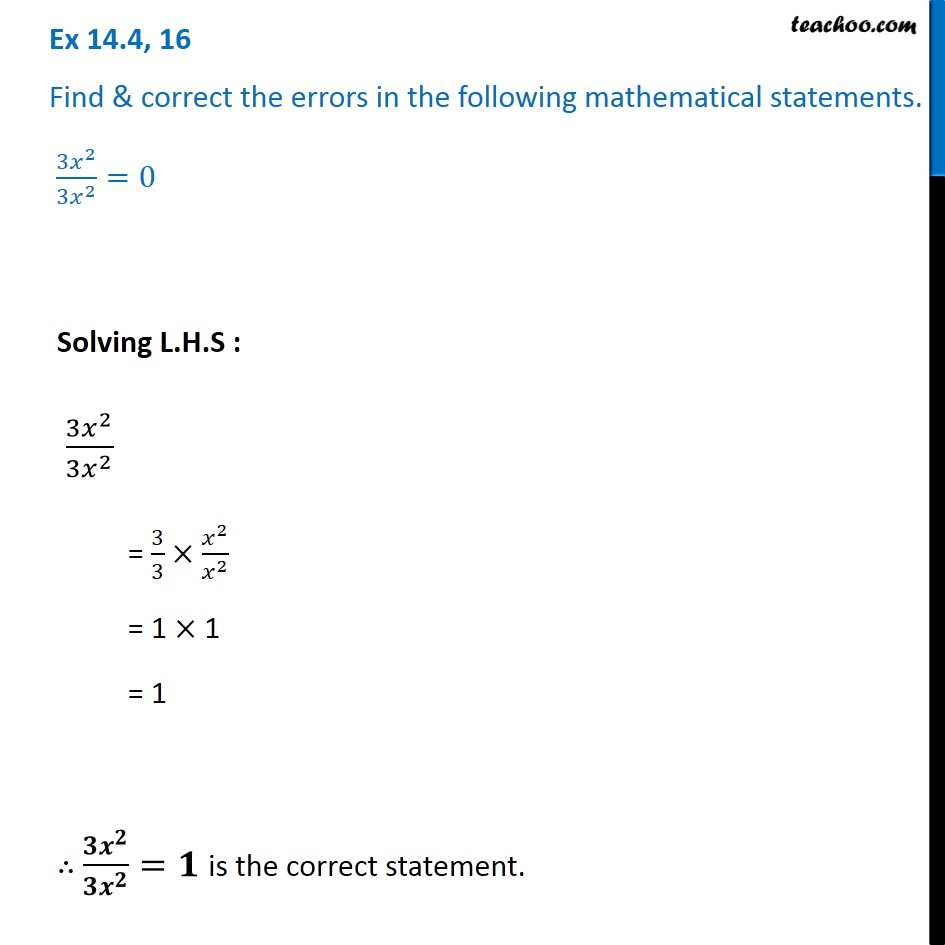Ex 14.4

Chapter 14 Class 8 Factorisation
Serial order wiseGet live Maths 1-on-1 Classs - Class 6 to 12

### Transcript

Ex 14.4, 16 Find & correct the errors in the following mathematical statements. 〖3𝑥〗^2/〖3𝑥〗^2 =0 Solving L.H.S : (3𝑥^2)/(3𝑥^2 ) = 3/3×𝑥^2/𝑥^2 = 1 × 1 = 1 ∴ (𝟑𝒙^𝟐)/(𝟑𝒙^𝟐 )=𝟏 is the correct statement.